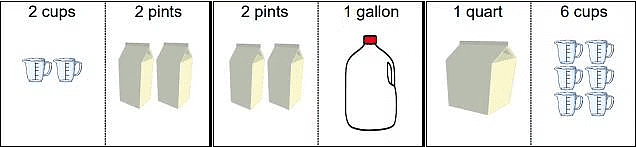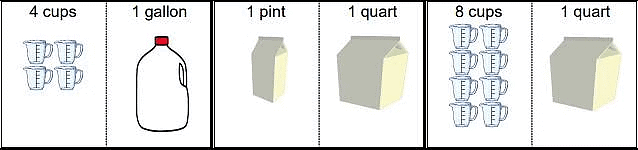Worksheet: Jugs And Mugs

# Worksheet: Jugs And Mugs - Notes | Study Mathematics for Class 3: NCERT - Class 3

 1 Crore+ students have signed up on EduRev. Have you?

Q.1. Which will you call as volume and which as capacity?

Q.2. Remember:

2 cups = 1 pint
4 cups = 2 pints = 1 quart
8 pints = 4 quarts = 1 gallon

Fill in the right numbers.
2 pints = _____ cups

Q.3. Fill in the gaps:
(i) 3500 ml = ............... litre ............... ml.
(ii) 2l, 300 ml = ............... millilitre.
(iii) Unit of measurement of kerosene oil is ............... .
(iv) Syrup is measured in ............... .

Q.4. Remember:

2 cups = 1 pint
4 cups = 2 pints = 1 quart
8 pints = 4 quarts = 1 gallon

Fill in the right numbers.
4 pints = _____ cups

Q.5. Subtract:
(i) 9 l 840 ml - 3 l 250 ml
(ii) 7 l 300 ml - 2 l 150 ml
(iii) 11 l 875 ml - 4 l 392 ml
(iv) 5 l 250 ml - 1 l 575 ml

Q.6. Remember:

2 cups = 1 pint
4 cups = 2 pints = 1 quart
8 pints = 4 quarts = 1 gallon

Fill in the right numbers.

2 quarts = _____ pints

Q.7. A milkman had 8 litre , 630 ml of milk. He sold 3 litre, 90 ml of it. How much milk is left unsold?

Q.8. Remember:

2 cups = 1 pint
4 cups = 2 pints = 1 quart
8 pints = 4 quarts = 1 gallon

Fill in the right numbers.
4 quarts = _____ pints

Q.9. A container contains 16 litre, 615 ml of water. 3 litre, 385 ml of more water can be poured in it. Find the capacity of the container.

Q.10. Remember:

2 cups = 1 pint
4 cups = 2 pints = 1 quart
8 pints = 4 quarts = 1 gallon

Fill in the right numbers.
2 quarts = _____ cups

Q.11. A bucket has 2 litters of capacity. I have a container of 200 ml. How many times will I use the container to fill the bucket?

Q.12. Remember:

2 cups = 1 pint
4 cups = 2 pints = 1 quart
8 pints = 4 quarts = 1 gallon

Fill in the right numbers.
4 quarts = _____ cups

(i) 5 litre 370 ml + 9 litre 850 ml
(ii) 4 litre 150 ml + 5 litre 810 ml
(iii) 7 litre 158 ml + 4 litre 275 ml
(iv) 7 litre 372 ml + 5 litre 640 ml

Q.14. Remember:

2 cups = 1 pint
4 cups = 2 pints = 1 quart
8 pints = 4 quarts = 1 gallon

Fill in the right numbers.
1 gallon = _____ pints

Q.15. There are two cows giving 7 litre, 670 ml and 6 litre, 250 ml of milk respectively. How much milk do the two cows give in all?

Q.16. Remember:

2 cups = 1 pint
4 cups = 2 pints = 1 quart
8 pints = 4 quarts = 1 gallon

Fill in the right numbers.
2 gallons = _____ quarts

Q.17. Which is more and by how much?
2 litre, 56 ml + 4 litre, 150 ml or 6 litre, 375 ml - 1 litre, 60 ml

Q.18. Which is more? Please circle.Q.19. Which is less? Please circle.The document Worksheet: Jugs And Mugs - Notes | Study Mathematics for Class 3: NCERT - Class 3 is a part of the Class 3 Course Mathematics for Class 3: NCERT.
All you need of Class 3 at this link: Class 3

## Mathematics for Class 3: NCERT

51 videos|84 docs|44 tests
 Use Code STAYHOME200 and get INR 200 additional OFF

## Mathematics for Class 3: NCERT

51 videos|84 docs|44 tests

Track your progress, build streaks, highlight & save important lessons and more!

,

,

,

,

,

,

,

,

,

,

,

,

,

,

,

,

,

,

,

,

,

;